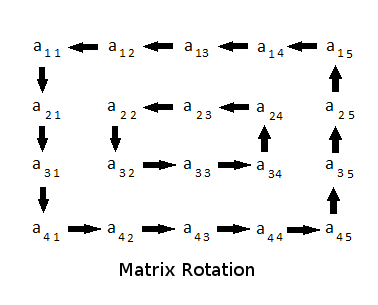## Matrix Layer Rotation

July 2017

This article analyses one of the HackerRank questions and solve it using simple data structures.

### Question

You are given a 2D matrix A (of dimension M×N) and a positive integer R. You have to rotate the matrix R times and print the resultant matrix. Rotation should be in the anti-clockwise direction.

Rotation of a 4×5 matrix is represented by the following figure. Note that in one rotation, you have to shift elements by one step only.

#### Example

Matrix A After rotating twice
```1    2   3   4
5    6   7   8
9   10  11  12
13  14  15  16```
```3   4   8  12
2  11  10  16
1   7   6  15
5   9  13  14 ```### Solution

The solution to this problem is fairly simple if proper data structures are used. It is straightforward to see that, the matrix should be broken down to circles (each rotating layer can be visualized as a circle) and each of the circles should be rotated R times. Now the problem simply breaks down to mapping the matrix (2D array) to a set of circles (circular arrays). At this point, the problem is half solved since it's just child's play to rotate elements of a circular array. Here, we implement circular arrays with 1D linear arrays with the help of modulus (%) operator. (LinearArray[i%l] will implement a circular array of length l, where the index is i.)Mapping ‘Example Matrix' to a set of Linear Arrays (representing circular arrays) will give you the following structure (Note: order of the element is at most important):

```Arr: [1, 2, 3, 4, 8, 12, 16, 15, 14, 13, 9, 5]
Arr: [6, 7, 11, 10]```

Now comes the most interesting thing, you don't have to actually rotate the array to print out the solution! Say you have functions get_loop_no(row, column) and get_element_pos(row, column) to identify the array number and index within the array for the element A[row, column]. To get the A[row, column] after rotation, all you have to do is to modify return value of get_element_pos() as:

`(get_element_pos() + no_rotations) % length_of_array`

The end